Processing ......FreeComputerBooks.com Free Computer, Mathematics, Technical Books and Lecture Notes, etc.

Combinatorics and Game TheoryRelated Book Categories:
•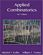Applied Combinatorics (Mitchel T. Keller, et al)

This is a text with more than enough material for a one-semester introduction to combinatorics. The original target audience was primarily computer science majors, but the topics included make it suitable for a variety of different students.

•Analytic Combinatorics (Philippe Flajolet and Robert Sedgewick)

The definitive treatment of analytic combinatorics. This self-contained text covers the mathematics underlying the analysis of discrete structures, with thorough treatment of a large number of applications.

•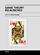Game Theory Relaunched (Hardy Hanappi)

This book collects recent research papers in game theory, which come from diverse scientific communities all across the world.

•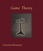Game Theory: An Open Access Textbook (Giacomo Bonanno)

This book is an introduction to game theory. Accessible to anybody with minimum knowledge of mathematics and no prior knowledge of game theory, yet it is also rigorous and includes several proofs.

•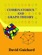An Introduction to Combinatorics and Graph Theory

This book walks the reader through the classic parts of Combinatorics and graph theory, while also discussing some recent progress in the area: on the one hand, providing material that will help students learn the basic techniques.

•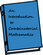Combinatorics Through Guided Discovery (Kenneth P. Bogart)

This book is an introduction to combinatorial mathematics, also known as combinatorics. The book focuses especially but not exclusively on the part of combinatorics that mathematicians refer to as 'counting'.

•Foundations of Combinatorics with Applications (Edward Bender)

This book of introduction to combinatorics is suitable for upper-level undergraduates and graduate students in engineering, science, and mathematics.

•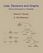Lists, Decisions and Graphs - With an Introduction to Probability

In this book, four basic areas of discrete mathematics are presented: Counting and Listing (Unit CL), Functions (Unit Fn), Decision Trees and Recursion (Unit DT), and Basic Concepts in Graph Theory (Unit GT).

•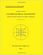Combinatorial Geometry with Application to Field Theory

Topics covered in this book include fundamental of combinatorics, algebraic combinatorics, topology with Smarandache geometry, combinatorial differential geometry, combinatorial Riemannian submanifolds, Lie multi-groups, etc.

•Notes on Convex sets, Polytopes, Polyhedra, Combinatorial Topology

This book may be viewed as a tutorial and a set of notes on convex sets, polytopes, polyhedra, combinatorial topology, Voronoi Diagrams and Delaunay Triangulations.

•LEDA: A Platform for Combinatorial and Geometric Computing

LEDA is a library of efficient data types and algorithms and a platform for combinatorial and geometric computing. This book, written by the main authors of LEDA, is the definitive account of how the system operates and how it can be used.

•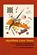Algorithmic Game Theory (Noam Nisan, Tim Roughgarden, et al)

This book covers many of the hottest area of useful new game theory research, introducing deep new problems, techniques, etc.

•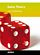Game Theory (Qiming Huang)

This book will give an impetus to the application of game theory to the modeling and analysis of modern communication, biology engineering, transportation, etc...

•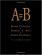A=B, by Marko Petkovsek, Herbert S. Wilf, et al

This book is of interest to mathematicians and computer scientists working in finite mathematics and combinatorics. It presents a breakthrough method for analyzing complex summations.

•Algebraic Combinatorics on Words (M. Lothaire)

This book is both a comprehensive introduction to the subject and a valuable reference source for researchers.

•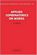Applied Combinatorics on Words (M. Lothaire)

The aim of this book is to present a unified treatment of some of the major fields of applications of combinatorics.

•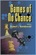Games of No Chance (Richard Nowakowski, editor)

This book deals with combinatorial games, that is, games not involving chance or hidden information.

•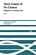More Games of No Chance (Richard J. Nowakowski)

This fascinating collection of articles by some of the top names in the field is a state-of-the-art look at combinatorial games.

•Games of No Chance 3 (Michael H. Albert, Richard J. Nowakowski)

This fascinating look at combinatorial games, that is, games not involving chance or hidden information, offers updates on standard games such as Go and Hex, on impartial games such as Chomp and Wythoff's Nim, etc.

•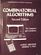Combinatorial Algorithms for Computers and Calculators (H. S. Wilf)

On one level, this is a collection of subroutines, in FORTRAN, for the solution of combinatorial problems.

•Greedy Algorithms ©2008 (Witold Bednorz)

This book covers fundamental, theoretical topics as well as advanced, practical applications of Greedy Algorithms.

•Multiagent Systems: Algorithmic, Game-Theoretic, and Logic, etc.

This comprehensive introduction to a burgeoning field is written from a computer science perspective, while bringing together ideas from operations research, game theory, economics, logic, and even philosophy and linguistics.

•Combinatorial and Computational Geometry (Jacob E. Goodman)

It includes surveys and research articles exploring geometric arrangements, polytopes, packing, covering, discrete convexity, geometric algorithms and their complexity, and the combinatorial complexity of geometric objects, particularly in low dimension.

•Architecture for Combinator Graph Reduction (Philip J. Koopman)

The results of cache-simulation experiments with an abstract machine for reducing combinator graphs are presented.

•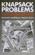Knapsack Problems: Algorithms and Computer Implementations

The text fully develops an algorithmic approach to Knapsack Problems without losing mathematical rigor.

•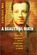A Beautiful Math: John Nash, Game Theory, and a Code of Nature

At the time of Nash's early work, game theory was briefly popular among some mathematicians and Cold War analysts.

•Games, Fixed Points and Mathematical Economics (C. Ewald)

This book gives the reader access to the mathematical techniques involved and goes on to apply fixed point theorems to proving the existence of equilibria for economics and for co-operative and noncooperative games.

•Game Theory: A Nontechnical Introduction

Striking an appropriate balance of mathematical and analytical rigor, this book teaches game theory by examples.

•Combinatorics and Game Theory

This is the previous page of Combinatorics and Game Theory, we are in the processing to convert all the books there to the new page. Please check this page daily!!!

Book CategoriesAll CategoriesRecent BooksIT Research LibraryMiscellaneous Books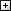Computer LanguagesComputer ScienceData Science/DatabasesElectronic EngineeringJava and Java EE (J2EE)Linux and UnixMathematicsMicrosoft and .NETMobile ComputingNetworking and CommunicationsSoftware EngineeringSpecial TopicsWeb Programming
Other Categories